# System of equations + percentages - math problems

#### Number of problems found: 79

• Together m+wWomen 30%. Men are 360 more. How many are together?
• Suit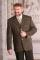The suit cost 119 euros. How much cost the pants if it is 50% cheaper than the jacket.
• Company and employeesThere are 370 employees in the company - women are 15% less than men. How many men work in the company?
• Comparing salariesSpouses compared salaries. Mrs. Janka found that a 20% higher salary than Mr Jaroslav. Together earned € 1,430. How much they earn each of them?
• Unknown number 10Number first increased by 30%, then by 1/5. What percentage we've increased the original number?
• Enterprise company30% of all employees in the enterprise are women. Men are 360 more. How many employees are in the company?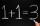Number 839 divide into the two addends that the first was 17 greater than 60% of the second. Determine these addends.
• Two workers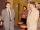Two workers make 138 parts/items. The first one produces 30% more than the second. How many items will each produce?
• Clothes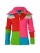CZK 2,400 cost clothes, a sweater by 150% than a T-shirt, trousers 2x more than a sweater, a jacket as a sweater and trousers together. What did the individual clothes cost?
• The city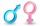In the city is 2/4 of women married to 3/4 men. What percentage of townspeople is single (not married)?
• ClassroomThere are eighty more girls in the class than boys. Boys are 40 percent and girls are 60 percent. How many are boys and how many girls?
• Tickets1260 tickets sold. On the first day, 80% was sold on the second day was sold. How many tickets were sold first and how much the next day?
• EmployeesThere are 1116 people working in three factory halls. In the first one, there are 18% more than the third, and 60 persons more than the second. How many employees work in individual halls?
• Crowns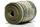1180 crowns is divided into three people that A got 20% less than B and C by 15% more than B. How many got A,B and C?
• Metal alloy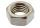What is the ratio of metals in the alloy that is in the 50 tonnes of steel to 30 kg nickel?
• Container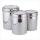First day got off 1/4 water from full container, second day 1/5 of the remaining water. How many percent remain container filled?
• RectangleThe width of the rectangle is 65% of its length. Perimeter of the rectangle is 132 cm. Determine the dimensions of the rectangle.
• RectangleFind the dimensions of the rectangle, whose perimeter is 108 cm and the length is 25% larger than the width.
• ContestantsThe three best contestants are to divide the total prize of CZK 4,200. The second gets 20% more than the third. And the first one gets 200 CZK less than the second and the third together. How much will everyone get?
• Dividing moneyThomas, Honza and Vasek are to divide 1220kč. Honza got 25% more than Thomas. Vasek 20% less than Thomas. How CZK each got?

Do you have an interesting mathematical word problem that you can't solve it? Submit a math problem, and we can try to solve it.

We will send a solution to your e-mail address. Solved examples are also published here. Please enter the e-mail correctly and check whether you don't have a full mailbox.

Please do not submit problems from current active competitions such as Mathematical Olympiad, correspondence seminars etc...